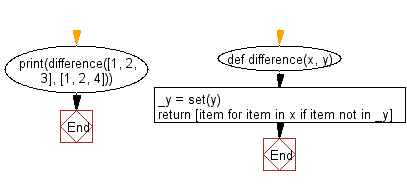﻿ Python: Calculate the difference between two iterables, without filtering duplicate values - w3resource# Python: Calculate the difference between two iterables, without filtering duplicate values

## Python List: Exercise - 247 with Solution

Write a Python program to calculate the difference between two iterables, without filtering duplicate values.

• Create a set from y.
• Use a list comprehension on x to only keep values not contained in the previously created set, _y.

Sample Solution:

Python Code:

``````def difference(x, y):
_y = set(y)
return [item for item in x if item not in _y]
print(difference([1, 2, 3], [1, 2, 4]))
```
```

Sample Output:

```
```

Flowchart:## Visualize Python code execution:

The following tool visualize what the computer is doing step-by-step as it executes the said program:

Python Code Editor:

Have another way to solve this solution? Contribute your code (and comments) through Disqus.

What is the difficulty level of this exercise?

Test your Python skills with w3resource's quiz

﻿

## Python: Tips of the Day

```print(2_000_000)
```2000000